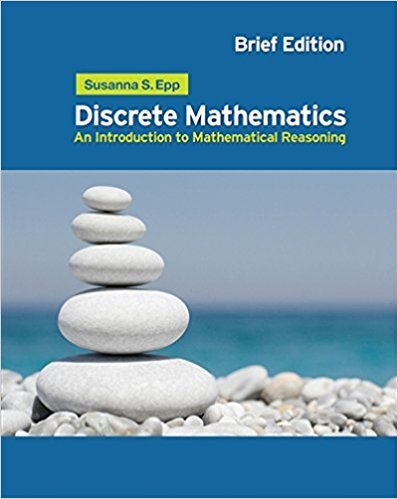×
Log in to StudySoup
Get Full Access to Math - Textbook Survival Guide
Join StudySoup for FREE
Get Full Access to Math - Textbook Survival Guide

Already have an account? Login here
×
Reset your password

Textbooks / Math / Discrete Mathematics: Introduction to Mathematical Reasoning 1

# Discrete Mathematics: Introduction to Mathematical Reasoning 1st Edition - Solutions by Chapter## Full solutions for Discrete Mathematics: Introduction to Mathematical Reasoning | 1st Edition

ISBN: 9780495826170Discrete Mathematics: Introduction to Mathematical Reasoning | 1st Edition - Solutions by Chapter

Solutions by Chapter
4 5 0 431 Reviews
##### ISBN: 9780495826170

Since problems from 10 chapters in Discrete Mathematics: Introduction to Mathematical Reasoning have been answered, more than 29646 students have viewed full step-by-step answer. Discrete Mathematics: Introduction to Mathematical Reasoning was written by and is associated to the ISBN: 9780495826170. This textbook survival guide was created for the textbook: Discrete Mathematics: Introduction to Mathematical Reasoning, edition: 1. The full step-by-step solution to problem in Discrete Mathematics: Introduction to Mathematical Reasoning were answered by , our top Math solution expert on 01/04/18, 08:37PM. This expansive textbook survival guide covers the following chapters: 10.

Key Math Terms and definitions covered in this textbook
• Big formula for n by n determinants.

Det(A) is a sum of n! terms. For each term: Multiply one entry from each row and column of A: rows in order 1, ... , nand column order given by a permutation P. Each of the n! P 's has a + or - sign.

• Cross product u xv in R3:

Vector perpendicular to u and v, length Ilullllvlll sin el = area of parallelogram, u x v = "determinant" of [i j k; UI U2 U3; VI V2 V3].

• Exponential eAt = I + At + (At)2 12! + ...

has derivative AeAt; eAt u(O) solves u' = Au.

• Free variable Xi.

Column i has no pivot in elimination. We can give the n - r free variables any values, then Ax = b determines the r pivot variables (if solvable!).

• Fundamental Theorem.

The nullspace N (A) and row space C (AT) are orthogonal complements in Rn(perpendicular from Ax = 0 with dimensions rand n - r). Applied to AT, the column space C(A) is the orthogonal complement of N(AT) in Rm.

• Gauss-Jordan method.

Invert A by row operations on [A I] to reach [I A-I].

• Hessenberg matrix H.

Triangular matrix with one extra nonzero adjacent diagonal.

• Least squares solution X.

The vector x that minimizes the error lie 112 solves AT Ax = ATb. Then e = b - Ax is orthogonal to all columns of A.

• Left nullspace N (AT).

Nullspace of AT = "left nullspace" of A because y T A = OT.

• Network.

A directed graph that has constants Cl, ... , Cm associated with the edges.

• Nilpotent matrix N.

Some power of N is the zero matrix, N k = o. The only eigenvalue is A = 0 (repeated n times). Examples: triangular matrices with zero diagonal.

• Pascal matrix

Ps = pascal(n) = the symmetric matrix with binomial entries (i1~;2). Ps = PL Pu all contain Pascal's triangle with det = 1 (see Pascal in the index).

• Plane (or hyperplane) in Rn.

Vectors x with aT x = O. Plane is perpendicular to a =1= O.

• Right inverse A+.

If A has full row rank m, then A+ = AT(AAT)-l has AA+ = 1m.

• Saddle point of I(x}, ... ,xn ).

A point where the first derivatives of I are zero and the second derivative matrix (a2 II aXi ax j = Hessian matrix) is indefinite.

• Semidefinite matrix A.

(Positive) semidefinite: all x T Ax > 0, all A > 0; A = any RT R.

• Singular matrix A.

A square matrix that has no inverse: det(A) = o.

• Toeplitz matrix.

Constant down each diagonal = time-invariant (shift-invariant) filter.

• Unitary matrix UH = U T = U-I.

Orthonormal columns (complex analog of Q).

• Vector space V.

Set of vectors such that all combinations cv + d w remain within V. Eight required rules are given in Section 3.1 for scalars c, d and vectors v, w.